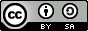## Tuesday, December 31, 2013

### Where I am on the Drake Equation at the end of 2013

I'm sure you are all familiar with the Drake Equation. It's straightforward: SETI scientist Frank Drake devised it as a way to estimate the number of alien civilizations in our galaxy that we might be able to detect. It's only intended to be a rough guide, and has survived the last 50 odd years because it serves as a good way to divvy up the questions we will need to resolve to answer the bigger question, "Are we alone"?

There are many fine explanations of the terms of the Drake Equation easily available to you, so I won't repeat them. Here are few:

This is how the equation is usually written:

N = R* • fp • ne • fl • fi • fc • L

As we move from left to right across the equation, the terms become less and less well known and harder to estimate, even when we know more. The only thing we're sure of with some of the terms is that none of them are zero, since we are here.

In the last few years, there has been progress. We have gone from knowing just the first term with any kind of accuracy ( a factor of 2 or so), to having solid estimates of the first three terms, and we can now begin to conceive of a research program that would give us an estimate of the fourth term.

Remember, we are only interested in rough numbers here: we just want to know, is N a lot or a little?

So, for 2013, I think we are here:

R = 10 stars/year (close enough)
fp = 1
ne = 0.1 planets/star (dominated by Red Dwarf stars, but not much less for sun like stars).
fl = 0.1 biospheres/planet(my guess, as are the rest of the terms, feel free to substitute your own)
fi = 0.1 sapients/biosphere (depends on how compelling you find the Dinosaur argument)
fc = 0.1 technological civilizations per sapient (pessimistic, in my view)
L = 1000 years (this is the "real" L, not just the "radio L")

Multiply the first six terms together, and you get 0.001 technological civilizations per year arising in our galaxy. After that, it all depends on L. For my estimate, L is only 1000 years, although some folks assume much larger numbers.  This gives the nice, neat and disappointing estimate of 1. That's what I'm going to call my 2013 number.

So, we need to talk about L. The other six terms could multiply up higher than I have here, but realistically aren't going to get much higher than 0.01 technological civilizations/year. As Shostak points out here, we are mostly just guessing, and the term isn't bounded like the other three unknown terms, which are at most 1 (but are probably less). If L is on the order of a century, then we very likely are alone in this galaxy. If civilizations find ways to propagate themselves to the stars and maintain their know-how and exploratory drive, then the number is more likely on the order of many millennia, then we should expect to have a few neighbors.